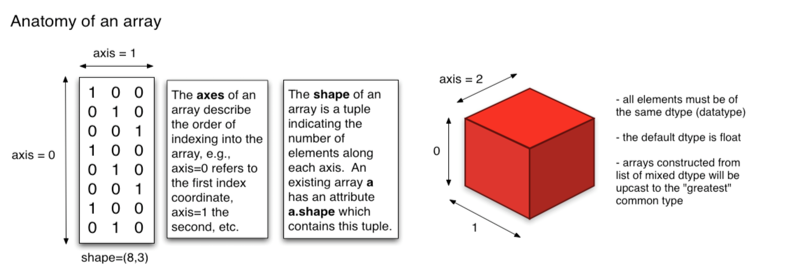# Reference

## argmax

``````>>> a = np.arange(6).reshape(2,3)
>>> a
array([[0, 1, 2],
[3, 4, 5]])
>>> np.argmax(a)
5
>>> np.argmax(a, axis=0)
array([1, 1, 1])
>>> np.argmax(a, axis=1)
array([2, 2])``````

## zeros

``````numpy.zeros(shape, dtype=float, order='C')
``````

# Topics

## Dimensions``````>>> a = np.ones((2, 3))
>>> a
array([[ 1.,  1.,  1.],
[ 1.,  1.,  1.]])
>>> np.sum(a, axis=0)
array([ 2.,  2.,  2.])
>>> np.sum(a, axis=1)
array([ 3.,  3.])
>>> np.sum(a, axis=1, keepdims=True)
array([[ 3.],
[ 3.]])
>>> np.sum(a, axis=1).shape
(2,)
>>> np.sum(a, axis=1, keepdims=True).shape
(2, 1)``````

## Slicing and Indexing

• `i:j:k` where `i` is the starting index, `j` is the stopping index, and `k` is the step (`k != 0`).
``````>>> x = np.array([0, 1, 2, 3, 4, 5, 6, 7, 8, 9])
>>> x[1:7:2]
array([1, 3, 5])``````
• if the number of objects in the selection tuple is less than N , then `:` is assumed for any subsequent dimensions.
• `Ellipsis` expand to the number of `:` objects needed to make a selection tuple of the same length as `x.ndim`. There may only be a single ellipsis present.
``````>>> x = np.array([[,,], [,,]])
>>> x.shape
(2, 3, 1)
>>> x[1:2]
array([[,
,
]])

>>> x[...,0]
array([[1, 2, 3],
[4, 5, 6]])``````
• Be careful that `x[1:2][3:4]` is not equivalent to `x[1:2, 3:4]`
• For the first one, both slicing(`1:2`, `3:4`) operates over the first dim, whereas for the second one, both slicing works on the first and second dim respectively.